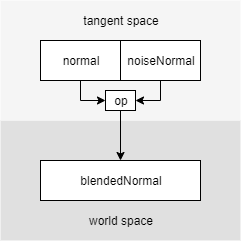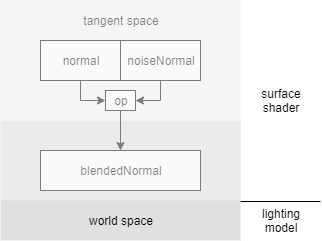# cg notes

kindergarten math and some cg notes

## Cross products encode sines

$$\mathbf{a} \times \mathbf{b} = \lVert \mathbf{a} \rVert \lVert \mathbf{b} \rVert \sin{(\theta)} \mathbf{n}$$

$$\mathbf{a}\times\mathbf{b} = (a_2 b_3 – a_3 b_2)\mathbf{i} + (a_3 b_1 – a_1 b_3)\mathbf{j} + (a_1 b_2 – a_2 b_1)\mathbf{k}$$

1. 叉积的模代表了两个向量围成平行四边形的面积，而平行四边形的面积很显然是 $\lVert \mathbf{a} \rVert \lVert \mathbf{b} \rVert \sin{(\theta)}$。
2. $\frac{\sqrt{(a_2 b_3 – a_3 b_2)^2 + (a_3 b_1 – a_1 b_3)^2 + (a_1 b_2 – a_2 b_1)^2}}{\lVert \mathbf{a} \rVert \lVert \mathbf{b} \rVert} = 1 – \frac{(a_1 b_1 + a_2 b_2 + a_3 b_3)^2}{(\lVert \mathbf{a} \rVert \lVert \mathbf{b} \rVert)^2} = 1 – \cos{(\theta)}^2$

## Unity 法线之旅struct Input
{
half4 tangentT; // 注意不能直接用 tangent，会报错
half3 normalW; // 也不能直接用 worldNormal，因为 o.Normal 被写了
...
}


struct SurfaceOutput {
half3 BlendedNormalW;
...
}


vert:

o.tangentT.xyz = UnityObjectToWorldDir(v.tangent.xyz);
o.tangentT.w = v.tangent.w; // specifies tangent direction
o.normalW = UnityObjectToWorldNormal(v.tangent.xyz);


surf:

half3 noiseNormal = tex2D(_NoiseTex, uv);
noiseNormal = 2.h * noiseNormal - 1.h;
half3 binormal = cross(IN.tangentT.xyz, IN.normalW) * IN.tangentT.w;
half3x3 rotation = half3x3(IN.tangentT.xyz, binormal, IN.normalW);
o.BlendedNormalW = mul(rotation, noiseNormal + o.Normal);


### 总结

vert v.normal object
surf IN.normal tangent
LightingModel s.Normal world

### TODO

• UNITY_INITIALIZE_OUTPUT
• TANGENT_SPACE_ROTATION
• worldNormal 的写入问题
• INTERNAL_DATA
• 怎么辨别法线所在的空间（基础）
• 怎么灵活转换各种空间的法线

5.15 修订

### 辨别

• tangent space normal 和普通的法线图长得一样
• object space normal 和世界法线差不多，呈现出十字形，但相对于物体固定
• world space normal 相对于世界坐标旋转

### 转换

tangent object world
tangent / tTw ⸰ wTo rotation
object oTw ⸰ wTt / UnityObjectToWorldNormal
world rotation^(-1) UnityWorldToObjectNormal /